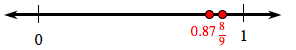### Home > MC1 > Chapter 9 > Lesson 9.2.5 > Problem9-114

9-114.

Copy the number line shown below and label the following numbers at their approximate place on the number line.1. $\frac { 1 } { 7 }$

1. $65\%$

1. $0.87$

1. $\frac { 8 } { 9 }$

For parts (a) through (d), try first estimating if the portions are greater than or less than one half ($50\%$ or $0.5$).Parts (c) and (d) are placed in their approximate place on the number line above. Can you place the portions in parts (a) and (b)?